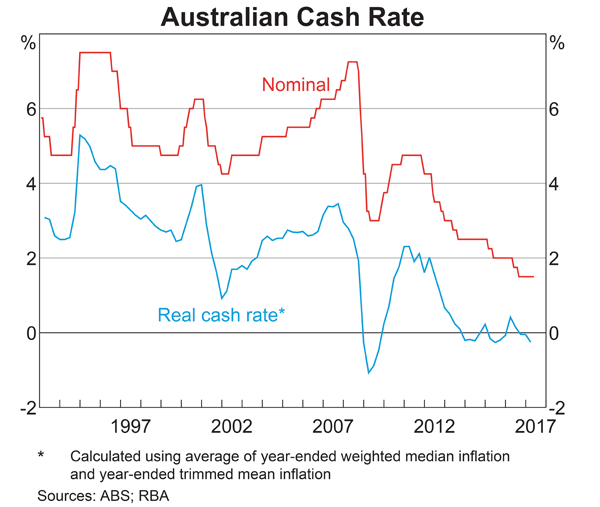# Fight Finance

#### CoursesTagsRandomAllRecentScores

A stock is expected to pay the following dividends:

 Cash Flows of a Stock Time (yrs) 0 1 2 3 4 ... Dividend ($) 0.00 1.00 1.05 1.10 1.15 ... After year 4, the annual dividend will grow in perpetuity at 5% pa, so; • the dividend at t=5 will be$1.15(1+0.05),
• the dividend at t=6 will be $1.15(1+0.05)^2, and so on. The required return on the stock is 10% pa. Both the growth rate and required return are given as effective annual rates. What will be the price of the stock in three and a half years (t = 3.5)? Most listed Australian companies pay dividends twice per year, the 'interim' and 'final' dividends, which are roughly 6 months apart. You are an equities analyst trying to value the company BHP. You decide to use the Dividend Discount Model (DDM) as a starting point, so you study BHP's dividend history and you find that BHP tends to pay the same interim and final dividend each year, and that both grow by the same rate. You expect BHP will pay a$0.55 interim dividend in six months and a $0.55 final dividend in one year. You expect each to grow by 4% next year and forever, so the interim and final dividends next year will be$0.572 each, and so on in perpetuity.

Assume BHP's cost of equity is 8% pa. All rates are quoted as nominal effective rates. The dividends are nominal cash flows and the inflation rate is 2.5% pa.

What is the current price of a BHP share?

 Portfolio Details Stock Expected return Standard deviation Correlation $(\rho_{A,B})$ Dollars invested A 0.1 0.4 0.5 60 B 0.2 0.6 140

What is the standard deviation (not variance) of the above portfolio?

You're considering making an investment in a particular company. They have preference shares, ordinary shares, senior debt and junior debt.

Which is the safest investment? Which will give the highest returns?

You just bought a house worth $1,000,000. You financed it with an$800,000 mortgage loan and a deposit of $200,000. You estimate that: • The house has a beta of 1; • The mortgage loan has a beta of 0.2. What is the beta of the equity (the$200,000 deposit) that you have in your house?

Also, if the risk free rate is 5% pa and the market portfolio's return is 10% pa, what is the expected return on equity in your house? Ignore taxes, assume that all cash flows (interest payments and rent) were paid and received at the end of the year, and all rates are effective annual rates.

Two companies BigDiv and ZeroDiv are exactly the same except for their dividend payouts.

BigDiv pays large dividends and ZeroDiv doesn't pay any dividends.

Currently the two firms have the same earnings, assets, number of shares, share price, expected total return and risk.

Assume a perfect world with no taxes, no transaction costs, no asymmetric information and that all assets including business projects are fairly priced and therefore zero-NPV.

All things remaining equal, which of the following statements is NOT correct?

You deposit cash into your bank account. Have you or debt?

Which of the below formulas gives the payoff $(f)$ at maturity $(T)$ from being short a call option? Let the underlying asset price at maturity be $S_T$ and the exercise price be $X_T$.

Below is the Australian central bank’s cash rate.From 2011 to 2017 the Australian central bank has implemented:

A firm has 20 million shares, earnings (or net income) of \$100 million per annum and a 60% debt-to-equity ratio where both the debt and asset values are market values rather than book values. Similar firms have a PE ratio of 12.

Which of the below statements is NOT correct based on a PE multiples valuation?